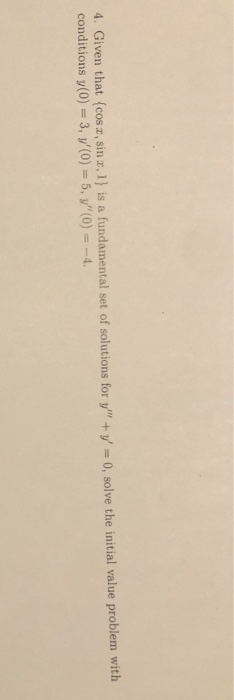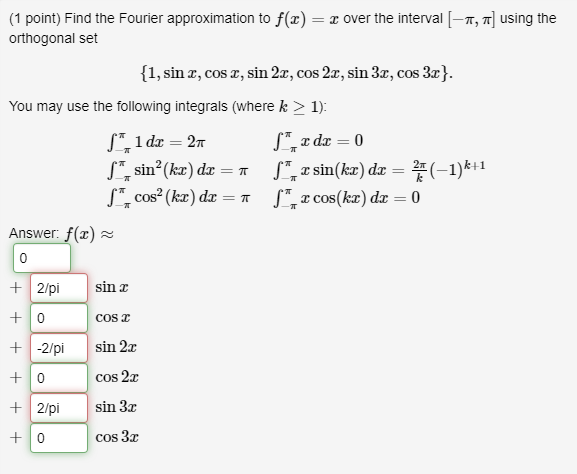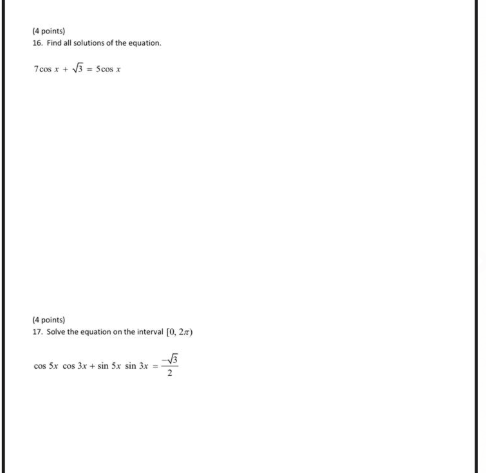# find all solutions in the interval [0,2 pi) sin(x+(3.14/3) + sin(x- 3.14/3) =1 sin^4 x cos^2 x Since sin (a+b) = sina cosb + cosb sina and sin (a-b) = sina cosb - cosb sina, the first problem can be written 2 sin x cos (pi/3)= sin x The soluti

find all solutions in the interval [0,2 pi)

sin(x+(3.14/3) + sin(x- 3.14/3) =1

sin^4 x cos^2 x

Since sin (a+b) = sina cosb + cosb sina
and
sin (a-b) = sina cosb - cosb sina,
the first problem can be written
2 sin x cos (pi/3)= sin x
The solution to sin x = 1 is x = pi/2

sin^4 cos^2 x = sin^4 x(1 - sin^2x)=0
The solutions are sin x = 0 and sin^2 x = 1. That would correspond to x=0, pi/2, pi, and 3 pi/4

This Homework Help Question: "find all solutions in the interval [0,2 pi) sin(x+(3.14/3) + sin(x- 3.14/3) =1 sin^4 x cos^2 x Since sin (a+b) = sina cosb + cosb sina and sin (a-b) = sina cosb - cosb sina, the first problem can be written 2 sin x cos (pi/3)= sin x The soluti" No answers yet.

We need 3 more requests to produce the answer to this homework help question. Share with your friends to get the answer faster!

0 /3 have requested the answer to this homework help question.

Once 3 people have made a request, the answer to this question will be available in 1-2 days.
All students who have requested the answer will be notified once they are available.
##### Add Answer of: find all solutions in the interval [0,2 pi) sin(x+(3.14/3) + sin(x- 3.14/3) =1 sin^4 x cos^2 x Since sin (a+b) = sina cosb + cosb sina and sin (a-b) = sina cosb - cosb sina, the first problem can be written 2 sin x cos (pi/3)= sin x The soluti
Similar Homework Help Questions
• ### Find all solutions on the interval [0,2pi] for the following: 2sin^2(x)-5sin(x)=-3 cos^2(x)+sin(x)=1

Find all solutions on the interval [0,2pi] for the following:2sin^2(x)-5sin(x)=-3cos^2(x)+sin(x)=1

• ### 2) Use the sum and difference identites sin[x + pi/4] + sin[x-pi/4] = -1 sinx cospi/4 + cosxsin pi/4 + sinx cos pi/4 - cosx sin pi/4 = -1 2 sin x cos pi/4 =-1 cos pi/4 = sqr2/2 2sin^x(sqrt2/2) = -1 sin x = -sqrt2 x = 7pi/4 and 5pi/4 Am I co

2) Use the sum and difference identites sin[x + pi/4] + sin[x-pi/4] = -1 sinx cospi/4 + cosxsin pi/4 + sinx cos pi/4 - cosx sin pi/4 = -1 2 sin x cos pi/4 =-1 cos pi/4 = sqr2/2 2sin^x(sqrt2/2) = -1 sin x = -sqrt2 x = 7pi/4 and 5pi/4 Am I correct?

• ### 4. Given that {cos x, sin 2, 1) is a fundamental set of solutions for y"...4. Given that {cos x, sin 2, 1) is a fundamental set of solutions for y" + y = 0, solve the initial value problem with conditions y(0) = 3, y'(0) = 5, "(0) = -4. 4. Given that {coso, sin x 1} is a fundamental set of solutions for y'" + y = 0, solve the initial value problem with conditions y(0) - 3, V'(0) -- 5. "(0) -4

• ### If a and B are two angles in Quadrant 2 such that tan a=-1/2 and tan B= -2/3, find cos(a+b)

If a and B are two angles in Quadrant 2 such that tan a=-1/2 and tan B= -2/3, find cos(a+b)?tan(a) = -1/2oppsite side = 1: adjacentside = 2hypotenuse = sqrt(1+4) = sqrt(5)sin(a) = 1/併5cos(a) = -2/併5tan(b) = -2/3opposite side = 2 and adjacentside = 3hypotenuse = sqrt(4+9) = 併13sin(b) = 2/併13cos(b) = -3/併13cos(a+b) = cosa cosb - sina sinb=(-2/併5)(-3/併13) - (1/併5)(2/併13)= 6/併65 - 2/併65= 4/併65right?

• ### find all solutions of the equation in the interval [0,2pi): cos(x)+sin(x)tan(x)=2

find all solutions of the equation in the interval [0,2pi): cos(x)+sin(x)tan(x)=2

• ### Find all values of x in the interval [0, 2 pi] that satisfy 2 cos x-1 = 0

Find all values of x in the interval [0, 2 pi] that satisfy 2 cos x-1 = 0.cos x = 1/2x = 60How do I find the other value of x?

• ### (1 point) Find the Fourier approximation to f(x) = x over the interval (-11, ] using...(1 point) Find the Fourier approximation to f(x) = x over the interval (-11, ] using the orthogonal set {1, sin , cos x, sin 22, cos 2x, sin 3%, cos 3x}. You may use the following integrals (where k > 1): | 1 dx = 27 - x dx = 0 sin(kx) dx = 1 L z sin(kx) dx = (-1)k+1 cos(kx) dx =1 L", cos(kx) dx = 0 Answer: f(2) + 2/pi sin + -2/pi + + 0...

• ### (4 points) 16. Find all solutions of the equation 7cos x + 3 = 500 (4...(4 points) 16. Find all solutions of the equation 7cos x + 3 = 500 (4 points) 17. Solve the equation on the interval [0, 2.) cos Sx cos 3x + sin 3x sin 3x =

• ### Determine the period, amplitude and phase shift for each given function: A)y = -4 cos 3x + 5 B)y = 2/3 sin (30x-90)-10 c)y = -0.38 tan (x/3+pi/3) d)y = pi cos(2x)+ pi

Determine the period, amplitude and phase shift for each given function: A)y = -4 cos 3x + 5B)y = 2/3 sin (30x-90)-10c)y = -0.38 tan (x/3+pi/3)d)y = pi cos(2x)+ pi

• ### Determine the period, amplitude and phase shift for each given function: A)y = -4 cos 3x + 5 B)y = 2/3 sin (30x-90)-10 c)y = -0.38 tan (x/3+pi/3) d)y = pi cos(2x)+ pi

Determine the period, amplitude and phase shift for each given function: A)y = -4 cos 3x + 5 B)y = 2/3 sin (30x-90)-10 c)y = -0.38 tan (x/3+pi/3) d)y = pi cos(2x)+ pi

Free Homework App